# Trigonometry + Heights & Distance

//Trigonometry + Heights & Distance

## Introduction to Trigonometry

Trigonometry words comes from Greek words (Trigonon + Metron). Which means measuring triangles.  As the name might suggest, is all about triangles.

Hence Trigonometry is one of the branches of mathematics which deals with the relationship between the sides of a triangle (right triangle) with its angles. There are 6 trigonometric functions for which the relation between sides and angles are defined – the 6 trigonometric functions are – sin, cos, tan, cosec, cot, sec

More specifically, trigonometry is about right-angled triangles, where one of the internal angles is 90°. Trigonometry is a system that helps us to work out missing or unknown side lengths or angles in a triangle.

## Basic Formulas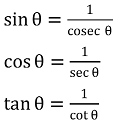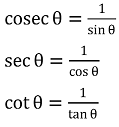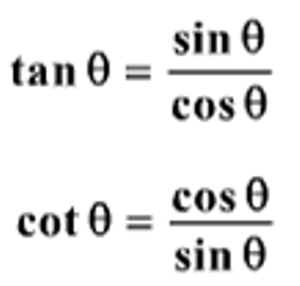## Best trick to Remember all the 30 Values of Trigonometric Functions ( त्रिकोणमितीय फलन ), watch the video below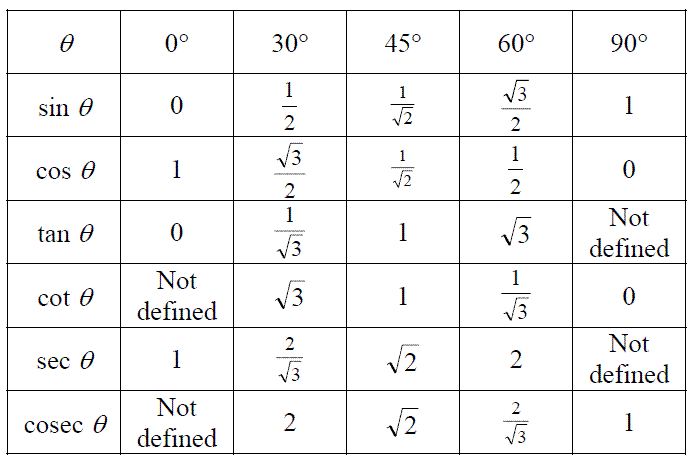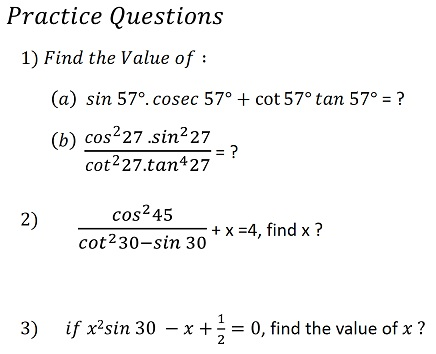• ### All Questions from SSC CGL Mains 2020 Exam – Solution

2021-05-22T08:26:57+05:30